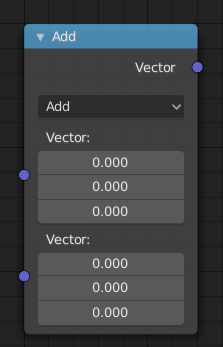# 矢量运算节点¶## 属性¶

A和B相加。

A和B之间的差值。

The entrywise product of A and B. $$(A.x * B.x, A.y * B.y, A.z * B.z)$$

The entrywise division of A by B. Division by zero returns zero. $$(A.x / B.x, A.y / B.y, A.z / B.z)$$

A和B的叉积。

A在B上的投影。

The reflection of A around the normal B. B need not be normalized.

A和B的点积。

A和B之间的距离。

A的长度

A与标量输入 缩放 相乘的结果。

Wrap

Wrap.

A的基面。

A的逐项取整。

A通过B逐项取模。

A的小数部分。

A的逐项取绝对值。

A和B中的输入最小值。

A和B的输入最大值。

Sine

The Sine of A.

Cosine

The Cosine of A.

Tangent

The Tangent of A.

## 输出选项¶

The output of the node is dynamic. It is either a vector or a scalar depending on the operator. For instance, the Length operator has a scalar output while the Add operator has a vector output.